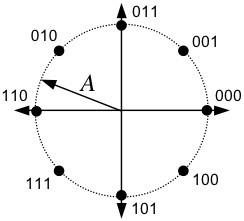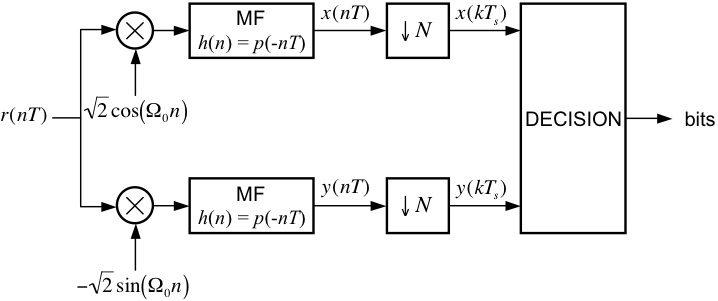EECS 690: M-ary Phase Shift Keying

Objective
The goal of this exercise is to correctly demodulate the noisy received signal in Rx8PSK.mat.

Signal Constellation

The transmitted data points are taken from the signal space constellation below.  The 8 points are equally spaced on a circle with radius A.
Each transmitted signal carries three information bits, as shown below.After being corrupted by noise, the received signal is demodulated by the system below, which detects the transmitted signal space points and outputs the estimated values of the transmitted bits.

Design Exercise

Part I: Generic MPSK TransmitterUsing blocks from the SIMULINK Block Library, the Signal Processing Blockset, and the Communications Blockset, design a MPSK modulator, patterned after the one shown above, to meet the following specifications:
Number of samples per symbol: 8
Normalized carrier frequency:    0.25 cycles/sample
Pulse shape:                            square-root raised cosine (SRRC) with roll-off = 0.5 and Lp = 6 symbols

As you can see, the above modulator is identical to the QPSK Modulator.  The only difference between modulators for M=4 and M=8 is the contents of the respective look-up tables.

Part II: Generic MPSK DetectorUsing blocks from the SIMULINK Block Library, the Signal Processing Blockset, and the Communications Blockset, design a MPSK detector, patterned after the one shown above, that is compatible with the modulator from Part I.  In your implementation, you might find it eaiser to output the signal space projections to the Matlab workspace and implement the decision logic in a post-processing fashion using a Matlab script.

[ Sanity Check ]
You can test your designs from Parts I and II by connecting the output of your modulator to the input of your detector.  The data source (Signal from Workspace block) emits symbol indexes, which are integers in the range 0 to 7.  Set this block to output the four-symbol sequence [0 2 4 6]'.  The I and Q look-up tables convert these indexes to the constellation points.  In your project window, go to the menu Simulation --> Configuration Parameters and set the parameters to:
Start Time:         0.0
Stop Time:          (4+12+1)*8
Solver options:    Type: Fixed-step, Solver: discrete (no continuous states)
Fixed step size:    1
Note: The 4 corresponds to the number of transmitted symbols, the 12 corresponds to the combined delay of the modulator pulse and the detector matched filter, the 1 corresponds to the delay of the downsample operation in the detector, and the 8 is the number of samples per symbol.

Remember, it is very important that your Downsample block is sampling at the correct "phase".  This may require some adjustment/experimentation on your part.  You want to sample the matched filter outputs when the magnitude of the value is at a maximum.  When you have successfully debugged your system, you are ready for the final part of the exercise.

Part III: Detecting an Unknown Data Set

Here are the steps for the final part of the exercise:
1. Connect the input of your detector to a From File block and set the filename to Rx8PSK.mat
2. Set the simulation parameters to:
Start Time:         0.0
Stop Time:          (98+12+1)*8
Solver options:    Type: Fixed-step, Solver: discrete (no continuous states)
Fixed step size:    1
3. Run the Simulation.
4. The last 98 values of the sampled matched filter outputs represent 42 ASCII characters.  Determine the message using your own conversion script or an ASCII table, such as the one found here.
5. E-mail your answer AND your detector model file (.mdl SIMULINK file) plus any additional Matlab code to esp "at" eecs.ku.edu.
6. Plot the eye diagram and signal space projections.  You do not need to submit these, but they are very helpful in understanding what is going on (as we shall soon see).

Back to the Simulink Exercises Page